# ILP Part 4 – Comparisons

This is the fourth part of the ILP series. For your convenience you can find other parts in the table of contents in Part 1 – Boolean algebra

We have already seen how to define Boolean algebra. We also know how to multiply variables or calculate division, remainder, powers, and roots. Today we are going to compare variables.

# Idea

Let’s assume that we have two integer variables: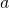and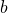. We want to know whetheris greater thanand we want to store this information in variable. We do not want to enforce this constraint, we only want to know the result of the comparison.
As we will see in later parts of this series, such a knowledge will allow us to calculate more sophisticated mathematical functions, like maximum or absolute value. But today we need to start with basics.

# Basics

First lets define variables: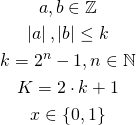We have two integer variables, both of them not greater than arbitrary defined number named. Since we will probably solve our models using computers, we assume thatis close to some power of two. We also define constant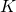, which we will use later. Finally, we define binary variable, which will hold the result of the comparison.

# Greater than comparison

Now we are going to implement greater than operator. We want to define the following: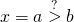which means thatis equal to one if and only ifis greater than, otherwiseis equal to zero. We can achieve this using the following constraints: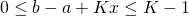Whenis greater than, then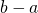is negative andmust be equal to one. Otherwise we would receive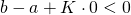which cannot happen because of the left inequality. However, whenis not greater than, their differenceis non-negative andhas to be zero.
We use valuegreather than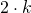because for case when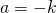and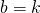, their difference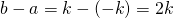. In that case we obtain: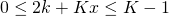Now imagine that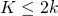. We get: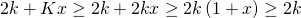On the other hand,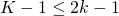, so inequality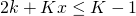has no solutions.

# Other operators

We have define only one relational operator, but now we are able to use it to define others. That is: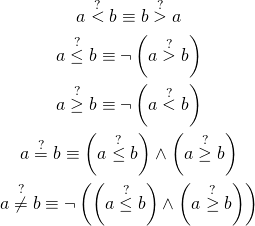# Summary

We defined relational operators and now we are able to compare variables. In the next part we will see how to utilize this knowledge to calculate absolute value, maximum, and minimum. These trivial functions are in fact really powerful and we will use them many times in other operators.